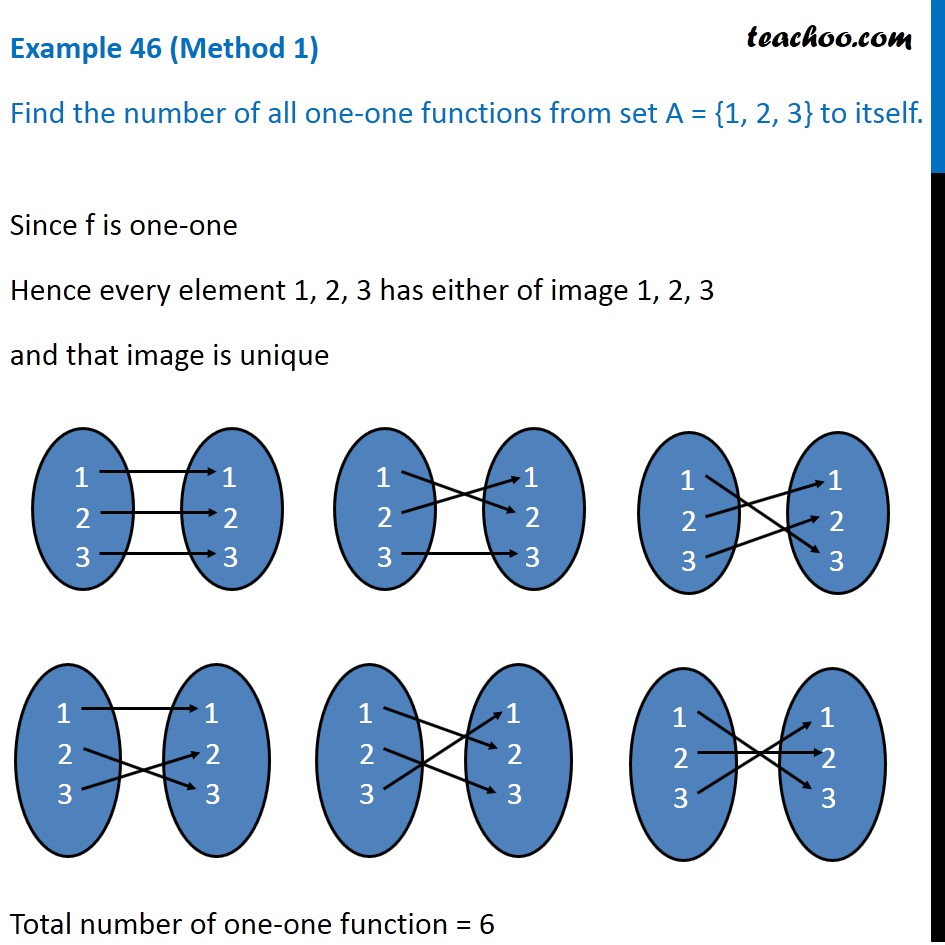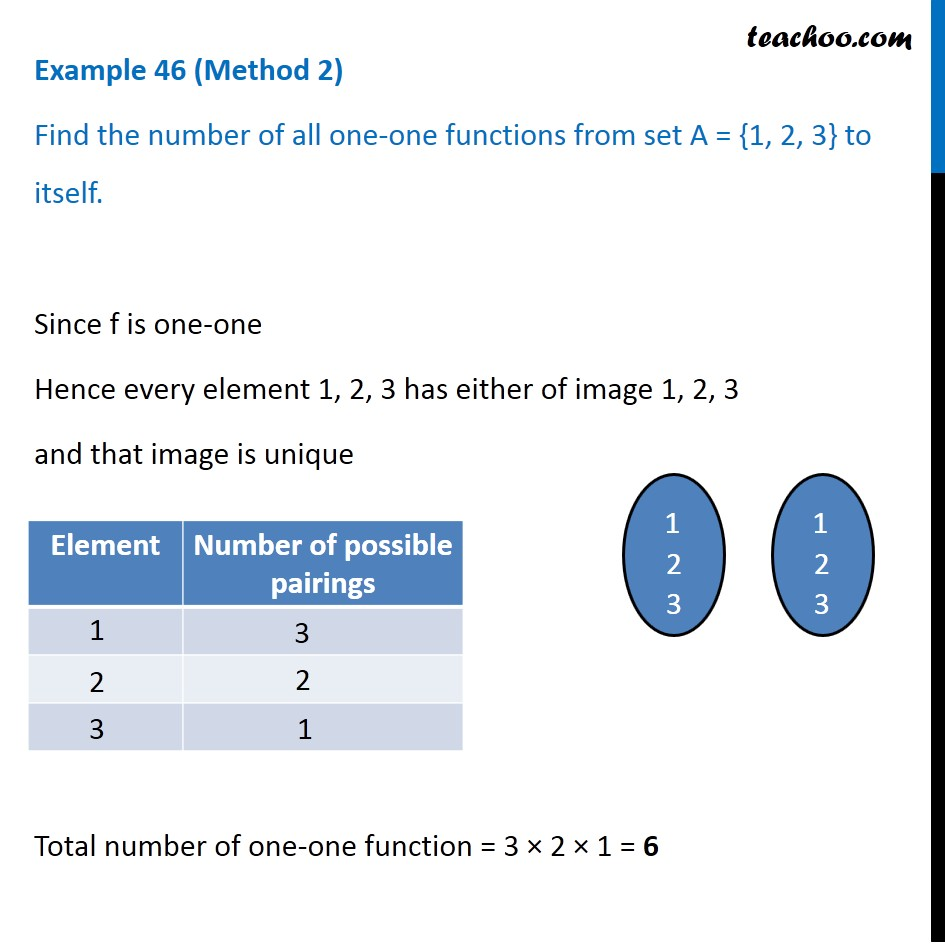Examples

Chapter 1 Class 12 Relation and Functions
Serial order wiseGet live Maths 1-on-1 Classs - Class 6 to 12

### Transcript

Example 46 (Method 1) Find the number of all one-one functions from set A = {1, 2, 3} to itself. Since f is one-one Hence every element 1, 2, 3 has either of image 1, 2, 3 and that image is unique Total number of one-one function = 6 Example 46 (Method 2) Find the number of all one-one functions from set A = {1, 2, 3} to itself. Since f is one-one Hence every element 1, 2, 3 has either of image 1, 2, 3 and that image is unique Total number of one-one function = 3 × 2 × 1 = 6